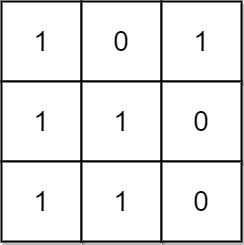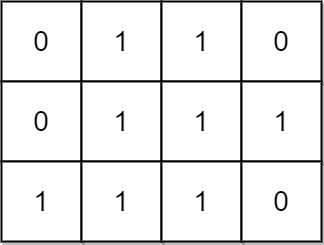# Count Submatrices With All Ones LeetCode Solution

Difficulty Level Medium

## Problem Statement

Count Submatrices With All Ones LeetCode Solution – We are given an m x n binary matrix and are asked to return the number of submatrices that have all ones.

## Examples and Explanations

Example 1:```Input: mat = [[1,0,1],[1,1,0],[1,1,0]]
Output: 13
Explanation:
There are 6 rectangles of side 1x1.
There are 2 rectangles of side 1x2.
There are 3 rectangles of side 2x1.
There is 1 rectangle of side 2x2.
There is 1 rectangle of side 3x1.
Total number of rectangles = 6 + 2 + 3 + 1 + 1 = 13.```

Example 2:```Input: mat = [[0,1,1,0],[0,1,1,1],[1,1,1,0]]
Output: 24
Explanation:
There are 8 rectangles of side 1x1.
There are 5 rectangles of side 1x2.
There are 2 rectangles of side 1x3.
There are 4 rectangles of side 2x1.
There are 2 rectangles of side 2x2.
There are 2 rectangles of side 3x1.
There is 1 rectangle of side 3x2.
Total number of rectangles = 8 + 5 + 2 + 4 + 2 + 2 + 1 = 24.```

## Approach

If we think about the brute force approach, the obvious idea that comes to our mind is to count the submatrices of all possible sizes that have ones. However, this approach will result in TLE. So, how can we improve this solution?

By solving dynamic programming we know that solving subproblems and then building solution upon them becomes easier than coming up with an entirely new strategy to tackle a problem. Even though, we are not implementing DP but will be using its strategy to count all submatrices having ones. Using the idea of DP, we will count all the submatrices that have a top left corner to some position.

Let’s create a submatrix of size a x b where a belongs to [0,m] & b belongs [0,n]. We will count all of the submatrices that have a top-left corner at that position. We can further use a variable bound to reduce the search space in the loop. Reducing the search space in the loop will always work because whenever the loop hit a zero, there will be no more submatrices to count, hence it will come out of the loop.

## Code

### C++ code for Count Submatrices With All Ones

```class Solution {
int countSubMatrices(vector<vector<int>>& mat, int a, int b) {
int r=mat.size(), c=mat.size();
int bound=c, count=0;
for(int i=a; i<r; i++) {
for(int j=b; j<bound; j++) {
if(mat[i][j]) count++;
else bound=j;
// cout<<i<<" "<<j<<" "<<bound<<" "<<count<<"\n";
}
}
return count;
}
public:
int numSubmat(vector<vector<int>>& mat) {
int r=mat.size(), c=mat.size();
int res=0;
for(int i=0; i<r; i++) {
for(int j=0; j<c; j++) {
res+=countSubMatrices(mat,i,j);
// cout<<"res = "<<res<<"\n";
}
}
return res;
}
};```

### Java code for Count Submatrices With All Ones

```class Solution {
public int countSubMatrices(int[][] mat, int a, int b) {
int r=mat.length, c=mat.length;
int bound=c, count=0;
for(int i=a; i<r; i++) {
for(int j=b; j<bound; j++) {
if(mat[i][j]==1) count++;
else bound=j;
}
}
return count;
}
public int numSubmat(int[][] mat) {
int r=mat.length, c=mat.length;
int res=0;
for(int i=0; i<r; i++) {
for(int j=0; j<c; j++) {
res+=countSubMatrices(mat,i,j);
}
}
return res;
}
}```

## Complexity Analysis for Count Submatrices With All Ones LeetCode Solution

• Time Complexity: O(m*m*n*n)
• creating submatrices of all sizes will take O(m*n)
• for every submatrix, another function is called that has 2 loops and in the worst time it takes O(m*n)
• Space Complexity: O(1)
• No extra space is required
Translate »